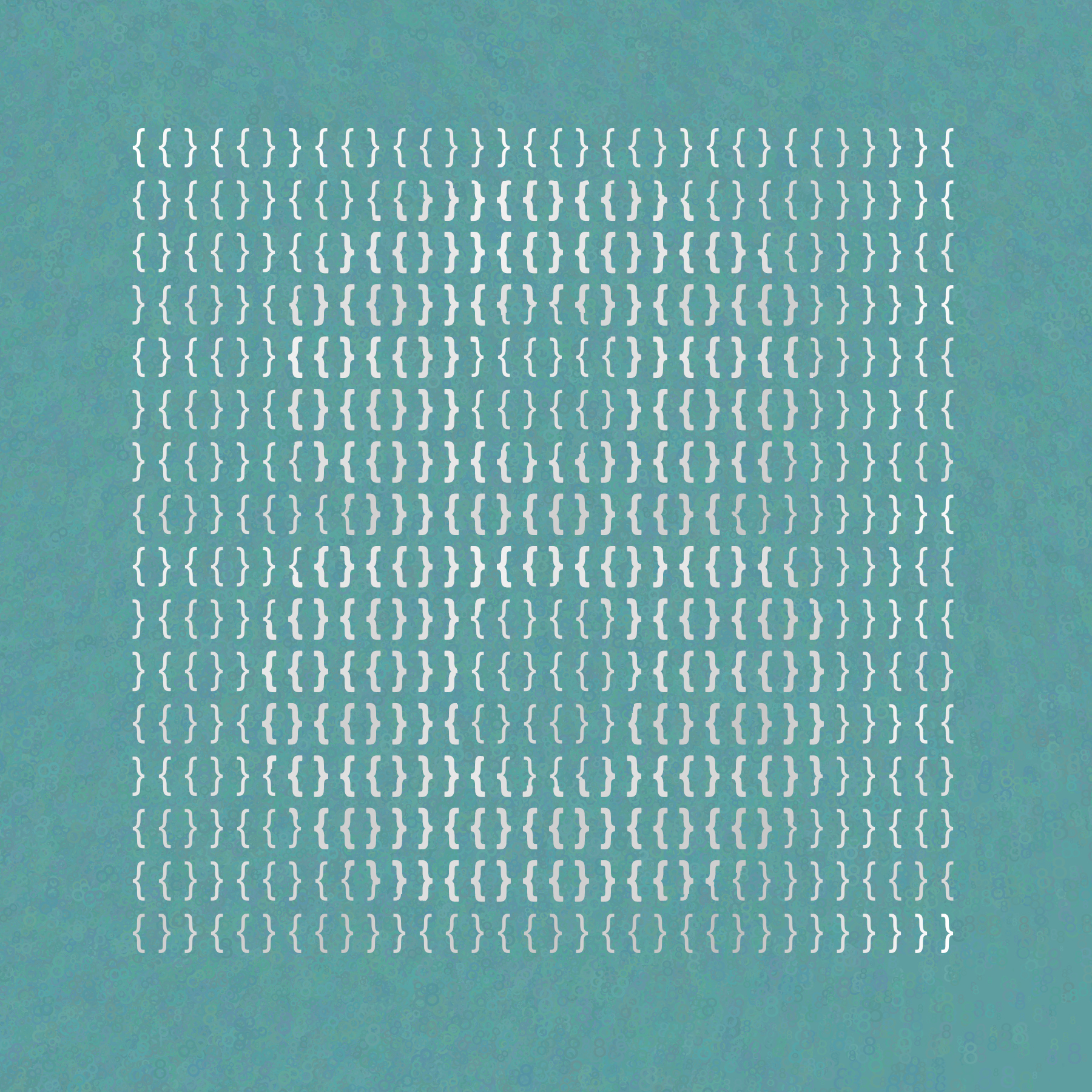# David Reimann

Professor of Mathematics and Computer Science
Albion College
Albion, Michigan, USA
Through my art I make visible the beauty and wonder I see in the often abstract world of mathematics. I enjoy giving visual representations to mathematical concepts such as number, form, and process. I often use patterns that convey messages at multiple levels and scales using a wide variety of mathematical elements and media. Some of my work contains fine detail that allows the art to be viewed differently depending on the distance between the viewer and the art. Another prevalent theme in my work is symmetry, where the overall pattern is created by repeated rotation or translation of similar units.Braces Of Eight
50 x 50 cm
Digital Print
2021
This artwork depicts the number eight using the set based construction of the non-negative integers described by John von Neumann around 1923. Juxtaposition of the symbols $$\{$$ and $$\}$$, rather than a comma, is used between set elements. The thickness of the symbols are varied to depict the numeral 8. The background texture is made from randomly sized, colored, and placed 8s. In this construction, zero is represented by the empty set: $$\{\}$$. Starting with $$0 = \{\}$$, we can define the integers recursively with the use of a successor function, $$s(n)$$, defined as follows: $s(n) = n + 1 = n \cup \{n\}.$ In general, the number $$n$$ is the set containing the numbers $$0$$ through $$n-1$$.